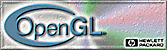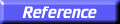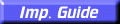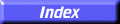# glDrawPixels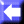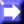glDrawPixels: write a block of pixels to the frame buffer.

C Specification | Parameters | Description | Errors | Associated Gets | See Also

##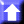C Specification

```void glDrawPixels(
GLsizei	 width,
GLsizei	 height,
GLenum	 format,
GLenum	 type,
const GLvoid *pixels)
```

##Parameters

width, height
Specify the dimensions of the pixel rectangle to be written into the frame buffer.
format
Specifies the format of the pixel data. Symbolic constants GL_COLOR_INDEX, GL_STENCIL_INDEX, GL_DEPTH_COMPONENT, GL_RGBA, GL_RED, GL_GREEN, GL_BLUE, GL_ALPHA, GL_RGB, GL_LUMINANCE, and GL_LUMINANCE_ALPHA are accepted.
type
Specifies the data type for pixels. Symbolic constants GL_UNSIGNED_BYTE, GL_BYTE, GL_BITMAP, GL_UNSIGNED_SHORT, GL_SHORT, GL_UNSIGNED_INT, GL_INT, and GL_FLOAT are accepted.
pixels
Specifies a pointer to the pixel data.

##Description

glDrawPixels reads pixel data from memory and writes it into the frame buffer relative to the current raster position. Use glRasterPos to set the current raster position; use glGet with argument GL_CURRENT_RASTER_POSITION to query the raster position.

Several parameters define the encoding of pixel data in memory and control the processing of the pixel data before it is placed in the frame buffer. These parameters are set with four commands: glPixelStore, glPixelTransfer, glPixelMap, and glPixelZoom. This reference page describes the effects on glDrawPixels of many, but not all, of the parameters specified by these four commands.

Data is read from pixels as a sequence of signed or unsigned bytes, signed or unsigned shorts, signed or unsigned integers, or single-precision floating-point values, depending on type. Each of these bytes, shorts, integers, or floating-point values is interpreted as one color or depth component, or one index, depending on format. Indices are always treated individually. Color components are treated as groups of one, two, three, or four values, again based on format. Both individual indices and groups of components are referred to as pixels. If type is GL_BITMAP, the data must be unsigned bytes, and format must be either GL_COLOR_INDEX or GL_STENCIL_INDEX. Each unsigned byte is treated as eight 1-bit pixels, with bit ordering determined by GL_UNPACK_LSB_FIRST (see glPixelStore).

width
height pixels are read from memory, starting at location pixels. By default, these pixels are taken from adjacent memory locations, except that after all width pixels are read, the read pointer is advanced to the next four-byte boundary. The four-byte row alignment is specified by glPixelStore with argument GL_UNPACK_ALIGNMENT, and it can be set to one, two, four, or eight bytes. Other pixel store parameters specify different read pointer advancements, both before the first pixel is read and after all width pixels are read. See the glPixelStore reference page for details on these options.

The widthheight pixels that are read from memory are each operated on in the same way, based on the values of several parameters specified by glPixelTransfer and glPixelMap. The details of these operations, as well as the target buffer into which the pixels are drawn, are specific to the format of the pixels, as specified by format. format can assume one of eleven symbolic values:

GL_COLOR_INDEX
Each pixel is a single value, a color index. It is converted to fixed-point format, with an unspecified number of bits to the right of the binary point, regardless of the memory data type. Floating-point values convert to true fixed-point values. Signed and unsigned integer data is converted with all fraction bits set to 0. Bitmap data convert to either 0 or 1.

Each fixed-point index is then shifted left by GL_INDEX_SHIFT bits and added to GL_INDEX_OFFSET. If GL_INDEX_SHIFT is negative, the shift is to the right. In either case, zero bits fill otherwise unspecified bit locations in the result.

If the GL is in RGBA mode, the resulting index is converted to an RGBA pixel with the help of the GL_PIXEL_MAP_I_TO_R, GL_PIXEL_MAP_I_TO_G, GL_PIXEL_MAP_I_TO_B, and GL_PIXEL_MAP_I_TO_A tables. If the GL is in color index mode, and if GL_MAP_COLOR is true, the index is replaced with the value that it references in lookup table GL_PIXEL_MAP_I_TO_I. Whether the lookup replacement of the index is done or not, the integer part of the index is then anded with 2b
1, where b is the number of bits in a color index buffer.

The GL then converts the resulting indices or RGBA colors to fragments by attaching the current raster position z coordinate and texture coordinates to each pixel, then assigning x and y window coordinates to the nth fragment such that

xn = xr + n mod width
yn = yr +n/widthwhere (xr, yr) is the current raster position. These pixel fragments are then treated just like the fragments generated by rasterizing points, lines, or polygons. Texture mapping, fog, and all the fragment operations are applied before the fragments are written to the frame buffer.

GL_STENCIL_INDEX
Each pixel is a single value, a stencil index. It is converted to fixed-point format, with an unspecified number of bits to the right of the binary point, regardless of the memory data type. Floating-point values convert to true fixed-point values. Signed and unsigned integer data is converted with all fraction bits set to 0. Bitmap data convert to either 0 or 1.

Each fixed-point index is then shifted left by GL_INDEX_SHIFT bits, and added to GL_INDEX_OFFSET. If GL_INDEX_SHIFT is negative, the shift is to the right. In either case, zero bits fill otherwise unspecified bit locations in the result. If GL_MAP_STENCIL is true, the index is replaced with the value that it references in lookup table GL_PIXEL_MAP_S_TO_S. Whether the lookup replacement of the index is done or not, the integer part of the index is then anded with 2b1, where b is the number of bits in the stencil buffer. The resulting stencil indices are then written to the stencil buffer such that the nth index is written to location

xn = xr + n mod width
yn = yr +n/widthwhere (xr, yr) is the current raster position. Only the pixel ownership test, the scissor test, and the stencil writemask affect these write operations.

GL_DEPTH_COMPONENT
Each pixel is a single-depth component. Floating-point data is converted directly to an internal floating-point format with unspecified precision. Signed integer data is mapped linearly to the internal floating-point format such that the most positive representable integer value maps to 1.0, and the most negative representable value maps to1.0. Unsigned integer data is mapped similarly: the largest integer value maps to 1.0, and 0 maps to 0.0. The resulting floating-point depth value is then multiplied by by GL_DEPTH_SCALE and added to GL_DEPTH_BIAS. The result is clamped to the range [0, 1].

The GL then converts the resulting depth components to fragments by attaching the current raster position color or color index and texture coordinates to each pixel, then assigning x and y window coordinates to the nth fragment such that

xn = xr + n mod width
yn = yr +n/widthwhere (xr, yr) is the current raster position. These pixel fragments are then treated just like the fragments generated by rasterizing points, lines, or polygons. Texture mapping, fog, and all the fragment operations are applied before the fragments are written to the frame buffer.

GL_RGBA
Each pixel is a four-component group: for GL_RGBA, the red component is first, followed by green, followed by blue, followed by alpha. Floating-point values are converted directly to an internal floating-point format with unspecified precision. Signed integer values are mapped linearly to the internal floating-point format such that the most positive representable integer value maps to 1.0, and the most negative representable value maps to1.0. (Note that this mapping does not convert 0 precisely to 0.0.) Unsigned integer data is mapped similarly: the largest integer value maps to 1.0, and 0 maps to 0.0. The resulting floating-point color values are then multiplied by GL_c_SCALE and added to GL_c_BIAS, where c is RED, GREEN, BLUE, and ALPHA for the respective color components. The results are clamped to the range [0, 1].

If GL_MAP_COLOR is true, each color component is scaled by the size of lookup table GL_PIXEL_MAP_c_TO_c, then replaced by the value that it references in that table. c is R, G, B, or A respectively.

The GL then converts the resulting RGBA colors to fragments by attaching the current raster position Z coordinate and texture coordinates to each pixel, then assigning x and y window coordinates to the nth fragment such that

xn = xr + n mod width
yn = yr +n/widthwhere (xr, yr) is the current raster position. These pixel fragments are then treated just like the fragments generated by rasterizing points, lines, or polygons. Texture mapping, fog, and all the fragment operations are applied before the fragments are written to the frame buffer.

GL_RED
Each pixel is a single red component. This component is converted to the internal floating-point format in the same way the red component of an RGBA pixel is. It is then converted to an RGBA pixel with green and blue set to 0, and alpha set to 1. After this conversion, the pixel is treated as if it had been read as an RGBA pixel.
GL_GREEN
Each pixel is a single green component. This component is converted to the internal floating-point format in the same way the green component of an RGBA pixel is. It is then converted to an RGBA pixel with red and blue set to 0, and alpha set to 1. After this conversion, the pixel is treated as if it had been read as an RGBA pixel.
GL_BLUE
Each pixel is a single blue component. This component is converted to the internal floating-point format in the same way the blue component of an RGBA pixel is. It is then converted to an RGBA pixel with red and green set to 0, and alpha set to 1. After this conversion, the pixel is treated as if it had been read as an RGBA pixel.
GL_ALPHA
Each pixel is a single alpha component. This component is converted to the internal floating-point format in the same way the alpha component of an RGBA pixel is. It is then converted to an RGBA pixel with red, green, and blue set to 0. After this conversion, the pixel is treated as if it had been read as an RGBA pixel.
GL_RGB
Each pixel is a three-component group: red first, followed by green, followed by blue. Each component is converted to the internal floating-point format in the same way the red, green, and blue components of an RGBA pixel are. The color triple is converted to an RGBA pixel with alpha set to 1. After this conversion, the pixel is treated as if it had been read as an RGBA pixel.
GL_LUMINANCE
Each pixel is a single luminance component. This component is converted to the internal floating-point format in the same way the red component of an RGBA pixel is. It is then converted to an RGBA pixel with red, green, and blue set to the converted luminance value, and alpha set to 1. After this conversion, the pixel is treated as if it had been read as an RGBA pixel.
GL_LUMINANCE_ALPHA
Each pixel is a two-component group: luminance first, followed by alpha. The two components are converted to the internal floating-point format in the same way the red component of an RGBA pixel is. They are then converted to an RGBA pixel with red, green, and blue set to the converted luminance value, and alpha set to the converted alpha value. After this conversion, the pixel is treated as if it had been read as an RGBA pixel.

The following table summarizes the meaning of the valid constants for the type parameter:

type Corresponding Type
GL_UNSIGNED_BYTE Unsigned 8-bit integer
GL_BYTE Signed 8-bit integer
GL_BITMAP Single bits in unsigned 8-bit integers
GL_UNSIGNED_SHORT Unsigned 16-bit integer
GL_SHORT Signed 16-bit integer
GL_UNSIGNED_INT Unsigned 32-bit integer
GL_INT 32-bit integer
GL_FLOAT Single-precision floating-point

The rasterization described so far assumes pixel zoom factors of 1. If glPixelZoom is used to change the x and y pixel zoom factors, pixels are converted to fragments as follows. If (xr, yr) is the current raster position, and a given pixel is in the nth column and mth row of the pixel rectangle, then fragments are generated for pixels whose centers are in the rectangle with corners at

(xr + zoomxn, yr + zoomy m)
(xr + zoomx (n + 1), yr + zoomy (m + 1))

where zoomx is the value of GL_ZOOM_X and zoomy is the value of GL_ZOOM_Y.

##Errors

• GL_INVALID_VALUE is generated if either width or height is negative.
• GL_INVALID_ENUM is generated if format or type is not one of the accepted values.
• GL_INVALID_OPERATION is generated if format is GL_RED, GL_GREEN, GL_BLUE, GL_ALPHA, GL_RGB, GL_RGBA, GL_LUMINANCE, or GL_LUMINANCE_ALPHA, and the GL is in color index mode.
• GL_INVALID_ENUM is generated if type is GL_BITMAP and format is not either GL_COLOR_INDEX or GL_STENCIL_INDEX.
• GL_INVALID_OPERATION is generated if format is GL_STENCIL_INDEX and there is no stencil buffer.
• GL_INVALID_OPERATION is generated if glDrawPixels is executed between the execution of glBegin and the corresponding execution of glEnd.

##Associated Gets

glGet with argument GL_CURRENT_RASTER_POSITION
glGet with argument GL_CURRENT_RASTER_POSITION_VALID

glAlphaFunc,
glBlendFunc,
glCopyPixels,
glDepthFunc,
glLogicOp,
glPixelMap,
glPixelStore,
glPixelTransfer,
glPixelZoom,
glRasterPos,